# Pyramid

A pyramid is a polyhedron with a polygonal base and triangular faces that meet at a point called an apex which is not in the plane of the polygonal base.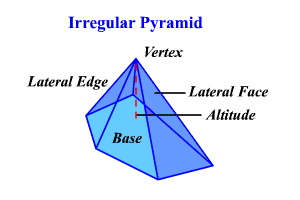Most of the pyramids that are studied in high school are regular pyramids .  These pyramids have the following characteristics:

• $1\right)$ The base is a regular polygon.
• $2\right)$ All lateral edges are congruent.
• $3\right)$ All lateral faces are congruent isosceles triangles.
• $4\right)$ The altitude meets the base at its center.

The altitude of a lateral face of a regular pyramid is the slant height.   In a non-regular pyramid, slant height is not defined.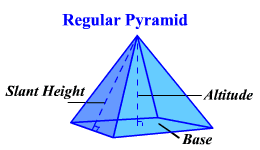## Lateral Surface Area

The lateral surface area of a regular pyramid is the sum of the areas of its lateral faces.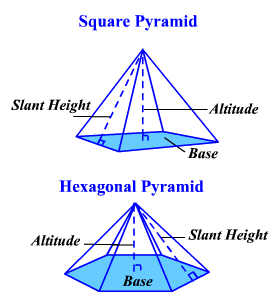The general formula for the lateral surface area of a regular pyramid is $L.S.A.=\frac{1}{2}pl$ where $p$ represents the perimeter of the base and $l$ the slant height.

Example 1:

Find the lateral surface area of a regular pyramid with a triangular base if each edge of the base measures $8$ inches and the slant height is $5$ inches.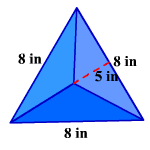The perimeter of the base is the sum of the sides.

$p=3\left(8\right)=24$ inches

$L.S.A.=\frac{1}{2}\left(24\right)\left(5\right)=60\text{\hspace{0.17em}}\text{\hspace{0.17em}}{\text{inches}}^{2}$

## Total Surface Area

The total surface area of a regular pyramid is the sum of the areas of its lateral faces and its base. The general formula for the total surface area of a regular pyramid is $T.S.A.=\frac{1}{2}pl+B$ where $p$ represents the perimeter of the base, $l$ the slant height and $B$ the area of the base.

Example 2:

Find the total surface area of a regular pyramid with a square base if each edge of the base measures $16$ inches, the slant height of a side is $17$ inches and the altitude is $15$ inches.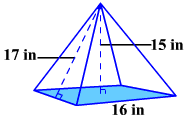The perimeter of the base is $4s$ since it is a square.

$p=4\left(16\right)=64$ inches

The area of the base is ${s}^{2}$ .

$B={16}^{2}=256\text{\hspace{0.17em}}\text{\hspace{0.17em}}{\text{inches}}^{2}$

$\begin{array}{l}T.S.A.=\frac{1}{2}\left(64\right)\left(17\right)+256\\ =544+256\\ =800\text{\hspace{0.17em}}\text{\hspace{0.17em}}{\text{inches}}^{2}\end{array}$

There is no formula for a surface area of a non-regular pyramid since slant height is not defined.  To find the area, find the area of each face and the area of the base and add them.

## Volume

The volume of a pyramid equals one-third the area of the base times the altitude (height) of the pyramid. $\left(V=\frac{1}{3}Bh\right)$ .

Example 3:

Find the volume of a regular square pyramid with base sides $10$ cm and altitude $12$ cm.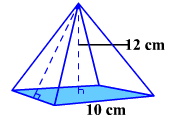$\begin{array}{l}V=\frac{1}{3}Bh\\ V=\frac{1}{3}{\left(10\right)}^{2}\left(12\right)=400\text{\hspace{0.17em}}\text{\hspace{0.17em}}{\text{cm}}^{2}\end{array}$